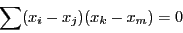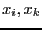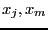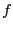Next: The SimplexConsistency procedure Up: Filtering simplification procedures Previous: The GlobalConsistencyTaylor procedure   Contents

## The SimpAngle procedure

This procedure may be used if one of the equation indicates that the scalar product of two vectors must have some given value. Hence such equation may be written as:where theare unknowns and theunknowns or constants.

A typical use of this procedure is given below, assuming that you have a list of equations EQ and a list of variable VAR:


eq1:=(x1-3)*(x2-4)+(y1-1)*(y2-4)-10:
SimpAngle([eq1],VAR,"SS"):
GeneralSolve(EQ,VAR,Init,"SS"):

Here the procedure generate the C++ procedure SS (which will be written in the file SS.C) and the solving algorithm GeneralSolve will use this procedure during the computation.

There is an optional last argument to this procedure which is a floating point number. If during the simplification there is change in the interval for a variable such that the width of the new range is lower by more thanfrom the width of the initial range, then the simplification process will be repeated until either the procedure detect that there is no solution to the system or that no change occur in the variable or that the amplitude of the change is lower than.Next: The SimplexConsistency procedure Up: Filtering simplification procedures Previous: The GlobalConsistencyTaylor procedure   Contents
Jean-Pierre Merlet 2012-12-20